# Resonance

Resonance in AC circuits implies a special frequency determined by the values of the resistance , capacitance , and inductance . For series resonance the condition of resonance is straightforward and it is characterized by minimum impedance and zero phase. Parallel resonance , which is more common in electronic practice, requires a more careful definition.

This is an active graphic. Click on either for more detail.Index

AC Circuits

 HyperPhysics***** Electricity and Magnetism R Nave
Go Back

# Series Resonance

The resonance of a series RLC circuit occurs when the inductive and capacitive reactances are equal in magnitude but cancel each other because they are 180 degrees apart in phase. The sharp minimum in impedance which occurs is useful in tuning applications. The sharpness of the minimum depends on the value of R and is characterized by the "Q" of the circuit.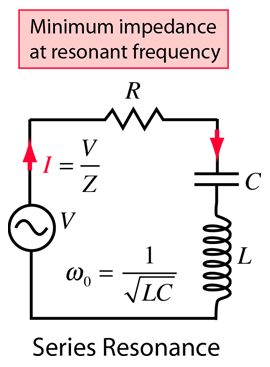Detailed expressions Calculation
Index

AC Circuits

 HyperPhysics***** Electricity and Magnetism R Nave
Go Back

# Selectivity and Q of a Circuit

Resonant circuits are used to respond selectively to signals of a given frequency while discriminating against signals of different frequencies. If the response of the circuit is more narrowly peaked around the chosen frequency, we say that the circuit has higher "selectivity". A "quality factor" Q, as described below, is a measure of that selectivity, and we speak of a circuit having a "high Q" if it is more narrowly selective.An example of the application of resonant circuits is the selection of AM radio stations by the radio receiver. The selectivity of the tuning must be high enough to discriminate strongly against stations above and below in carrier frequency, but not so high as to discriminate against the "sidebands" created by the imposition of the signal by amplitude modulation.
 The selectivity of a circuit is dependent upon the amount of resistance in the circuit. The variations on a series resonant circuit at right follow an example in Serway & Beichner. The smaller the resistance, the higher the "Q" for given values of L and C. The parallel resonant circuit is more commonly used in electronics, but the algebra necessary to characterize the resonance is much more involved.Using the same circuit parameters, the illustration at left shows the power dissipated in the circuit as a function of frequency. Since this power depends upon the square of the current, these resonant curves appear steeper and narrower than the resonance peaks for current above. The quality factor Q is defined by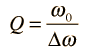where Δω is the width of the resonant power curve at half maximum.

Since that width turns out to be Δω =R/L, the value of Q can also be expressed as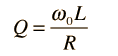The Q is a commonly used parameter in electronics, with values usually in the range of Q=10 to 100 for circuit applications.

Index

AC Circuits

Reference
Serway & Beichner
Ch 33

 HyperPhysics***** Electricity and Magnetism R Nave
Go Back

# Power in a Series Resonant Circuit

The average power dissipated in a series resonant circuit can be expressed in terms of the rms voltage and current as follows: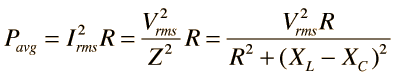Using the forms of the inductive reactance and capacitive reactance, the term involving them can be expressed in terms of the frequency.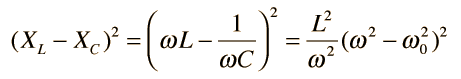where use has been made of the resonant frequency expressionSubstitution now gives the expression for average power as a function of frequency.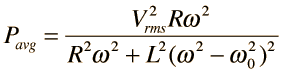This power distribution is plotted at left using the same circuit parameters as were used in the example on the Q factor of the series resonant circuit The average power at resonance is just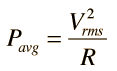since at the resonant frequency ω0 the reactive parts cancel so that the circuit appears as just the resistance R.
Index

AC Circuits

Reference
Serway & Beichner
Ch 33

 HyperPhysics***** Electricity and Magnetism R Nave
Go Back